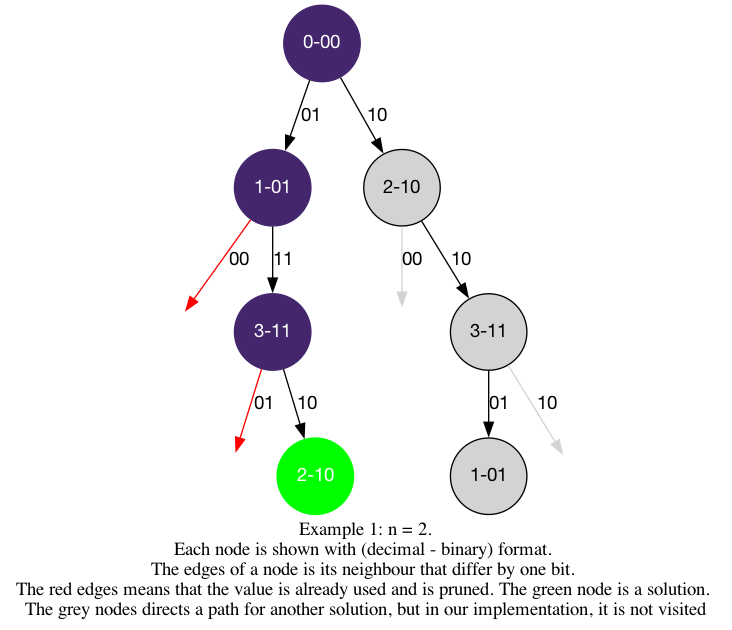# LeetCode Grey Code Solution

An n-bit gray code sequence is a sequence of `2n` integers where:

• Every integer is in the inclusive range `[0, 2n - 1]`,
• The first integer is `0`,
• An integer appears no more than once in the sequence,
• The binary representation of every pair of adjacent integers differs by exactly one bit, and
• The binary representation of the first and last integers differs by exactly one bit.

Given an integer `n`, return any valid n-bit gray code sequence.

Example 1:

Input: `n = 2`

Output: `[0,1,3,2]`

Explanation:

The binary representation of `[0,1,3,2]` is `[00,01,11,10]`.

• `00` and `01` differ by one bit
• `01` and `11` differ by one bit
• `11` and `10` differ by one bit
• `10` and `00` differ by one bit

`[0,2,3,1]` is also a valid gray code sequence, whose binary representation is `[00,10,11,01]`.

• `00` and `10` differ by one bit
• `10` and `11` differ by one bit
• `11` and `01` differ by one bit
• `01` and `00` differ by one bit

Example 2:

Input: `n = 1`

Output: `[0,1]`

Constraints:

• `1 <= n <= 16`

## Solution

In this question, we are dealing with binary representation of integers, we will be using bit manipulation. To learn more, checkout Bitmask Intro.

The two important operations we will use are the shift operator (`<<`) and the XOR (exclusive or) operator (`^`).

• `1 << i`: will shift `1` by `i` bits to the left, the new binary number will have `i` zeros after a `1`.
• `code ^ (1 << i)`: flips the `i`th bit (from the right) in `code`'s binary representation. By the property of XOR that `0^1 = 1` and `1^1 = 0`.

Now, we can use these two operations to find the candidates for the next code. And since we are only looking for one solution, we can use the aggregation template to return earlier. We will apply the backtracking2 template and fill in the logic.

• `is_leaf`: `start_index == 2**n`, we have used all integers
• `get_edges`: `new_code = code ^ (1 << i)` where `0 <= i < n`, this `new_code` has one flipped bit on the `i`-th bit, it can potentially be the next code
• `is_valid`: `new_code` is valid when we have not used it (`not in visited`)

#### Implementation

``````1def grayCode(self, n: int) -> List[int]:
2    length = 1 << n   # same as 2**n
3    visited = [False] * length
4
5    def dfs(start_index, code):
6        if start_index == length:
7            return True
8        for i in range(n):
9            new_code = code ^ (1 << i)
10            print(new_code)
11            if not visited[new_code]:
12                path.append(new_code)
13                visited[new_code] = True
14                if dfs(start_index+1, new_code): return True
15                visited[new_code] = False
16                path.pop()
17        return False
18
19    path = 
20    visited = True
21    dfs(1, 0)
22    return path``````